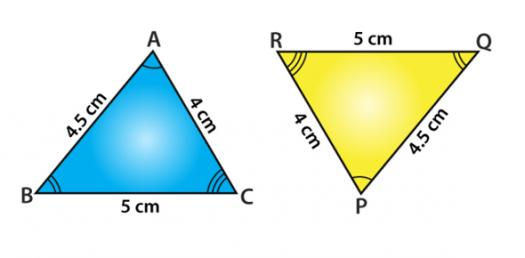# Spherical Geometry

14 Questions | Total Attempts: 2008Settings.

• 1.
Spherical Geometry is an example of non-Euclidean geometry.
• A.

True

• B.

False

• 2.
Parallel lines can be drawn on a sphere.
• A.

True

• B.

False

• 3.
When two lines intersect on a sphere ________ angles are made.
• 4.
The sum of the angles of a triangle on a sphere can be at most _____________ degrees.
• 5.
The dotted line on the sphere below is called a great circle.
• A.

True

• B.

False

• 6.
Vertical angles are congruent in both Euclidean and spherical geometry.
• A.

True

• B.

False

• 7.
Which statement is not true in spherical geometry.
• A.

Two lines that are perpendicular to the same line are parallel to each other.

• B.

A great circle is finite and returns to its original starting point.

• C.

An arc of a great circle is the shortest path between two points.

• D.

There is a unique great circle passing through any pair of nonpolar points.

• 8.
A line in spherical geometry has infinite length.
• A.

True

• B.

False

• 9.
Infinitely many great circles can be drawn through the poles on a sphere.
• A.

True

• B.

False

• 10.
The shortest distance on a sphere is a(n) ________________.
• A.

Great circle

• B.

Sphere

• C.

Arc of a great circle

• D.

Triangle

• 11.
A triangle can have more than one right angle.
• A.

True

• B.

False

• 12.
Perpendicular lines on a sphere form how many right angles?
• A.

6

• B.

4

• C.

8

• 13.
On a sphere, two lines intersect at one and only one point.
• A.

True

• B.

False

• 14.
All lines of longitude are great circles.
• A.

True

• B.

False

Related TopicsBack to top